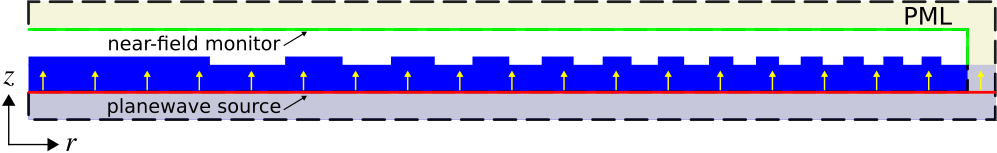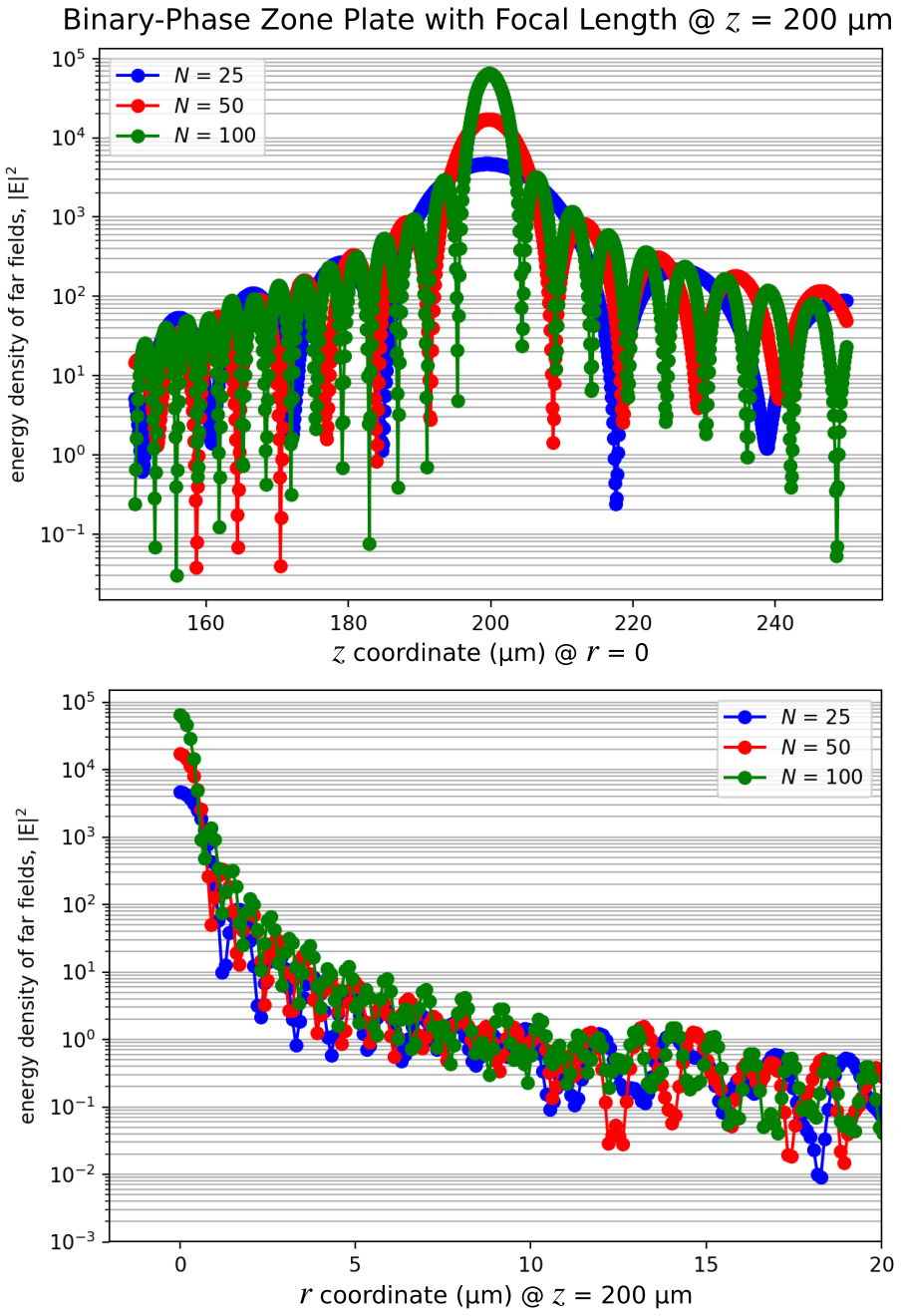# Focusing Properties of a Binary-Phase Zone Plate¶

It is also possible to compute a near-to-far field transformation in cylindrical coordinates. This is demonstrated in this example for a binary-phase zone plate which is a rotationally-symmetric diffractive lens used to focus a normally-incident planewave to a single spot.

Using scalar theory, the radius of the $n$th zone can be computed as:

$$r_n^2 = n\lambda (f+\frac{n\lambda}{4})$$

where $n$ is the zone index (1,2,3,...,$N$), $f$ is the focal length, and $\lambda$ is the operating wavelength. The main design variable is the number of zones $N$. The design specifications of the zone plate are similar to the binary-phase grating in Tutorial/Mode Decomposition/Diffraction Spectrum of a Binary Grating with refractive index of 1.5 (glass), $\lambda$ of 0.5 μm, and height of 0.5 μm. The focusing property of the zone plate is verified by the concentration of the electric-field energy density at the focal length of 0.2 mm (which lies outside the cell). The planewave is incident from within a glass substrate and spans the entire length of the cell in the radial direction. The cell is surrounded on all sides by PML. A schematic of the simulation geometry for a design with 25 zones and flat-surface termination is shown below. The near-field line monitor is positioned at the edge of the PML.In [ ]:
import meep as mp
import numpy as np
import math
import matplotlib.pyplot as plt

resolution = 25             # pixels/μm

dpml = 1.0                  # PML thickness
dsub = 2.0                  # substrate thickness
zh = 0.5                    # zone-plate height
zN = 25                     # number of zones (odd zones: π phase shift, even zones: none)
focal_length = 200          # focal length of zone plate
spot_length = 100           # far-field line length
ff_res = 10                 # far-field resolution

pml_layers = [mp.PML(thickness=dpml)]

wvl_cen = 0.5
frq_cen = 1/wvl_cen
dfrq = 0.2*frq_cen

## ref: eq. 7 of http://zoneplate.lbl.gov/theory
r = [math.sqrt(n*wvl_cen*(focal_length+n*wvl_cen/4)) for n in range(1,zN+1)]

cell_size = mp.Vector3(sr,0,sz)

sources = [mp.Source(mp.GaussianSource(frq_cen,fwidth=dfrq,is_integrated=True),
component=mp.Er,
center=mp.Vector3(0.5*sr,0,-0.5*sz+dpml),
size=mp.Vector3(sr)),
mp.Source(mp.GaussianSource(frq_cen,fwidth=dfrq,is_integrated=True),
component=mp.Ep,
center=mp.Vector3(0.5*sr,0,-0.5*sz+dpml),
size=mp.Vector3(sr),
amplitude=-1j)]

glass = mp.Medium(index=1.5)

geometry = [mp.Block(material=glass,
size=mp.Vector3(sr,0,dpml+dsub),
center=mp.Vector3(0.5*sr,0,-0.5*sz+0.5*(dpml+dsub)))]

for n in range(zN-1,-1,-1):
geometry.append(mp.Block(material=glass if n % 2 == 0 else mp.vacuum,
size=mp.Vector3(r[n],0,zh),
center=mp.Vector3(0.5*r[n],0,-0.5*sz+dpml+dsub+0.5*zh)))

sim = mp.Simulation(cell_size=cell_size,
boundary_layers=pml_layers,
resolution=resolution,
sources=sources,
geometry=geometry,
dimensions=mp.CYLINDRICAL,
m=-1)

## near-field monitor
n2f_obj = sim.add_near2far(frq_cen, 0, 1, mp.Near2FarRegion(center=mp.Vector3(0.5*(sr-dpml),0,0.5*sz-dpml),size=mp.Vector3(sr-dpml)))

sim.run(until_after_sources=100)

ff_r = sim.get_farfields(n2f_obj, ff_res, center=mp.Vector3(0.5*(sr-dpml),0,-0.5*sz+dpml+dsub+zh+focal_length),size=mp.Vector3(sr-dpml))
ff_z = sim.get_farfields(n2f_obj, ff_res, center=mp.Vector3(z=-0.5*sz+dpml+dsub+zh+focal_length),size=mp.Vector3(z=spot_length))

E2_r = np.absolute(ff_r['Ex'])**2+np.absolute(ff_r['Ey'])**2+np.absolute(ff_r['Ez'])**2
E2_z = np.absolute(ff_z['Ex'])**2+np.absolute(ff_z['Ey'])**2+np.absolute(ff_z['Ez'])**2

plt.figure(dpi=200)
plt.subplot(1,2,1)
plt.semilogy(np.linspace(0,sr-dpml,len(E2_r)),E2_r,'bo-')
plt.xlim(-2,20)
plt.xticks([t for t in np.arange(0,25,5)])
plt.grid(True,axis="y",which="both",ls="-")
plt.xlabel(r'$r$ coordinate (μm)')
plt.ylabel(r'energy density of far fields, |E|$^2$')
plt.subplot(1,2,2)
plt.semilogy(np.linspace(focal_length-0.5*spot_length,focal_length+0.5*spot_length,len(E2_z)),E2_z,'bo-')
plt.grid(True,axis="y",which="both",ls="-")
plt.xlabel(r'$z$ coordinate (μm)')
plt.ylabel(r'energy density of far fields, |E|$^2$')
plt.suptitle(r"binary-phase zone plate with focal length $z$ = {} μm".format(focal_length))
plt.tight_layout()
plt.savefig("zone_plate_farfields.png")


Note that the volume specified in get_farfields via center and size is in cylindrical coordinates. These points must therefore lie in the $\phi = 0$ ($rz = xz$) plane. The fields $E$ and $H$ returned by get_farfields can be thought of as either cylindrical ($r$,$\phi$,$z$) or Cartesian ($x$,$y$,$z$) coordinates since these are the same in the $\phi = 0$ plane (i.e., $E_r=E_x$ and $E_\phi=E_y$). Also, get_farfields tends to gradually slow down as the far-field point gets closer to the near-field monitor. This performance degradation is unavoidable and is due to the larger number of $\phi$ integration points required for accurate convergence of the integral involving the Green's function which diverges as the evaluation point approaches the source point.

Shown below is the far-field energy-density profile around the focal length for both the r and z coordinate directions for three lens designs with $N$ of 25, 50, and 100. The focus becomes sharper with increasing $N$ due to the enhanced constructive interference of the diffracted beam. As the number of zones $N$ increases, the size of the focal spot (full width at half maximum) at $z = 200$ μm decreases as $1/\sqrt{N}$ (see eq. 17 of the reference). This means that doubling the resolution (halving the spot width) requires quadrupling the number of zones.